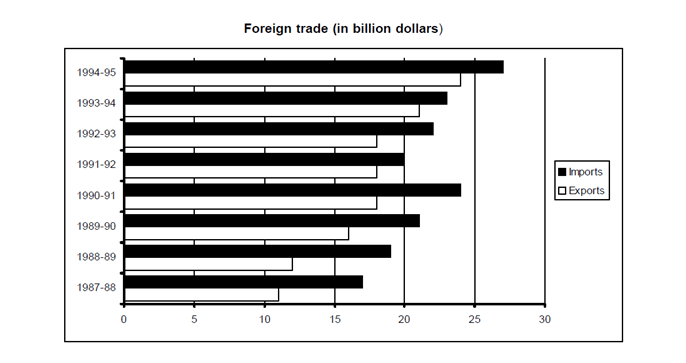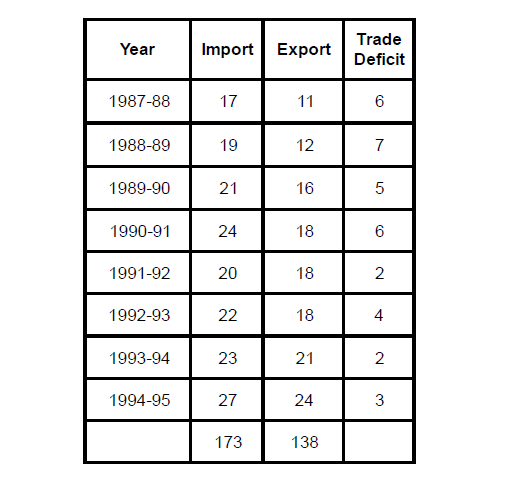Directions for question 1 to 4: Follow the bar graph and answer the following questionQuestion 1.  In which year out of the following was the trade deficit lowest?
a. 1987-88
b. 1988-89
c. 1990-91
d. 1993-94

Question 2. In how many years was the trade deficit more than the trade deficit in the succeeding year?
a. 1
b. 2
c. 3
d. 4

Question 3. Export earnings in 1991-92 is how many percent of imports in 1992-93?
a. 82%
b. 85%
c. 81.81%
d. 15%

Question 4. In the last 2 years the total export earnings have accounted for how many per cent of the value of the imports?
a. 80%
b. 88%
c. 95%
d. 90%

By analyzing the data in the given bar graph, we can construct the following table:We have calculated the trade deficit for the given years in the table above using the formula:

Trade deficit = Imports – Exports

∴By analyzing the table we can say that Trade Deficitis lowest for the year 1993-94 and for years 1991-92, i.e. 2 billion dollars. Hence, out of the given options, 1993-94 becomes the right answer.

From the table we can conclude that trade deficit is more than the trade deficit in the succeeding years in 1988-89, 1990-91, 1992 -93 i.e.for 3 years.

From the table above we know the following:

Export earnings in 1991-92 = 18 billion dollars

Import earnings in 1992-93 = 22 billion dollars

Required percentage = 18/22× 100= 81.81%